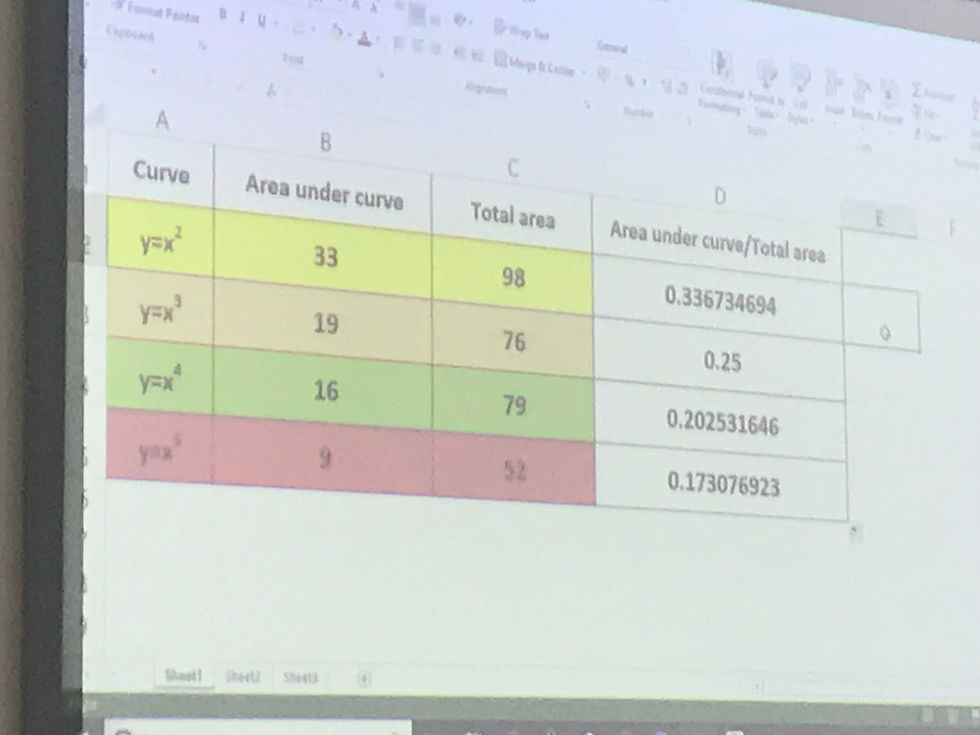top of page
Search
• PixiMaths

# More TAM fun

Every TAM experience I have makes me further question the way in which I teach A Level maths. I’m not saying all my lessons lower down the school are fun, but they’re definitely more engaging and interactive than what I usually do with year 12. In Birmingham earlier this week, I was stretched, challenged, questioned and questioned myself too, on approaches, teaching styles, course content and how best to support students to make progress. On Monday we focused largely on integration. We were asked to cut up graphs of y=x^2, y=x^3, y=x^4 and y=x^5 along the axes leaving only the positive quadrant, then along lines of our choosing that were parallel to the aces and met at the graph, to create a rectangle. Then came the scales! Yes, seriously. Thankfully it wasn’t just me that pulled a face at this, as I looked around the room to see other equally perplexed faces. All the y=x^2 rectangles were weighed together, then the y=x^3 rectangles, and so on. And their weights in grams recorded. Next we had to cut along the graphs, disposing of the piece above the graph and keeping the bit below? See where this is going? Then we weighed all the y=x^2 areas-under-the-graphs together, then the y=x^3 areas, etc. Again, the weights were recorded.An underlying focus of the two days was conjecture and generalisation, so at this point in the task we were asked what we thought. Considering y=x with an area under the graph being half of the area of the rectangle, my group and I wondered if the area under the graph would be 1/(n+1), where n is the order of the graph. The results looked like this:Now obviously, thinking about it afterwards, of course there would be this ratio relationship, because we know that integration is given by S y dx = x^(n+1)/n+1, but we had great fun getting there and now had a much stronger understanding of how integration works. I'm not sure if I'm going to look at this with my own class yet; I think it largely depends on how quickly I can get through the A Level content.